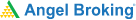# Glossary of Using Options Greeks1. Delta : Measure of option price sensitivity to changes in stock price.
2. Gamma: Measure of option price sensitivity to changes in Delta.
3. Theta: Measures the time decay of an option as we move towards expiry.
4. Vega: Measures the sensitivity of the option price to changes in volatility.
5. Volatility: It is used in option pricing formulas to gauge the fluctuations in the returns of the underlying assets.
6. Options: Contracts that give the right to buy or sell an amount of an underlying asset at a predetermined price before the contract expires.
7. Call Option: This gives the holder the right to buy a stock
8. Put Option: This gives the holder the right to sell a stock.
9. Daily Volatility Formula: The formula for daily volatility is computed by finding out the square root of the variance of a daily stock price. Daily Volatility formula = √Variance

Learning & Earning is now super simple₹ 0 Equity Delivery

No Hidden Charges₹ 20 Per Order For Intraday

FAQ,Currencies & CommoditiesZERO Brokerage*

on ALL SegmentsFREE Margin

Trade Funding

1. Debit: the amount of money you paid to purchase a new long position, or the amount of money paid to close an existing short position.
2. Defined Risk: This is a situation where you know precisely the maximum amount of money you can lose in any trade or spread.
3. In-the-money (ITM): this refers to the strike price of the option relative to the current market price of the underlying.
4. Out-of-the-money (OTM): this refers to the strike price of the option relative to the current market price of the underlying.
5. Spread: any position that involves a combination of two or more unique options.
6. Theta Decay: This describes the natural tendency for the value of options to lose a little bit of value every day.
7. Daily Volatility Formula: The formula for daily volatility is computed by finding out the square root of the variance of a daily stock price. Daily Volatility formula = √Variance
8. Annualized Volatility Formula: Annualized Volatility Formula = √252 * √Variance
9. Historical volatility: It is based on past and concrete data, institutional investors follow a thumb rule when calculating it. It is measured using a variance.

Comments (0)

Add Comment

Get Information Mindfulness!

Catch-up With Market

News in 60 Seconds.The perfect starter to begin and stay tuned with your learning journey anytime and anywhere.Ready To Trade? Start withOpen an account